American Journal of Life Sciences
Volume 3, Issue 5, October 2015, Pages: 345-351

The United Equation of Animal Growth

Alexey A. Zotin

Laboratory of Evolutional Developmental Biology, Kol’tsov Institute of Developmental Biology of The Russian Academy of Sciences, Moscow, Russia

Email address:

aazotin@mail.ru

To cite this article:

Alexey A. Zotin. The United Equation of Animal Growth.American Journal of Life Sciences.Vol.3, No. 5, 2015, pp. 345-351. doi: 10.11648/j.ajls.20150305.12

Abstract: The equation that can describe all known types of animal growth is proposed. The equation contains two basic coefficients, one of which determines the initial rate of growth; the other determines the change of the growth rate. In separate cases two correction coefficients should be taken into account, one of which is connected with morphogenetic processes and the other is connected with spontaneous decrease of the body weight. Biorhythms that accompany growth process can be described by the equation as well. Linear recursive form of the equation allows to use regression analysis and, therefore, comparative intrapopulation, interpopulation and interspecies analysis of growth. Examples of approximation by the equation of own and literature experimental data are shown. Values of the coefficients of the equation for different types of growth in different stages of ontogenesis of the various animal systematic groups are calculated. The equation is suitable to describe change of any biological parameter which is associated with body weight by allometric (power) dependence.

Keywords: Body Weight Changes, Developmental Biology, Growth, Mathematical Model

Contents

1. Introduction

Growth is one of the main processes occurring during animal development. Therefore, it is not surprising that its description is a subject of many studies [1-9].

All the observed growth curves can be divided into two broad categories [10,11].

1. Infinite growth. The size of the animal is permanently increased. Such growth is observed in the majority of poikilothermic animals.

2. Finite growth. The size of the animal first reach maximum and then continuously decrease. Such growth is characteristic for some poikilothermic and for most homoeothermic animals.

Each of these categories can be divided by several types depending on the shape of growth curve: exponential, parabolic, linear, decelerating and S-shaped. It should be noted that the types of curves are not limited by the above types. Cases of absence of weight change or even degradation of body weight are often observed for some ontogenetic periods. Usually, these changes are not considered as growth [3,4]. I believe that such point of view is not entirely true, because result of growth depends on the balance between processes of assimilation and dissimilation. Thus, cases of weight decreasing can be considered as "negative" growth when dissimilation prevails over the assimilation. The problem of quantitative description of the growth is complicated by the fact that, as a rule, is accompanied by biorhythms, having both exogenous and endogenous nature [12,13].

At first view, it is impossible to find a single equation that can describe all observed types of growth. Large number of equations was proposed, which more or less well describe different growth curves . However, a single equation that can describe all of the observed growth curves still has not been found.

Nevertheless, detailed analysis of the dependence of the body weight or length (M) on age (t) indicated that at least for the infinite growth only three basic equation can be used .

1. The exponential equation:(1)

All the coefficients of this equation are positive.

2. Parabolic equation: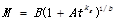(2)

An important special case of (2) is a linear equation when the coefficients kg and b equal 1.

3. The asymptotic equation. This equation is called the von Bertalanffy growth equation and is used most often: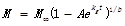(3)

kg < 0; all other coefficients are positive. If 0 < b ≤ 1, then (3) describes the asymptotic decelerating growth type (convex curve without inflection). If b > 1, (3) describes the asymptotic S-shaped growth type with one point of inflection. To calculate the values of the coefficients of (3) one often uses recurrent form of this equation [15,16]: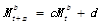(4)

Mt is growth parameter at the age t; Mt+m is growth parameter at the age t + m; b is constant from Bertalanffy equation (3); m is the time interval between measurements.

It appears that all these equations can be reduced to one, which describes not only an infinite growth, but all types of observed growth curves. Deduction and analysis of such united equation is an aim of this article.

2. Methods

The equations (1)-(4) were used as a basis for deduction of united growth equation. The possibility of the united growth equation to fit experimental data was tested on own and literature experimental data. Coefficients of the united equation were determined by methods of regression analysis. The suitability of the equation is evaluated by the criterion of nonlinearity .

Fitting of data by united equation and the construction of the time series were performed by program Matlab (version 7.3.0.267, developed by The MathWorks, Inc, USA). To distinguish main trend and rhythmic processes from the time series singular spectrum analysis were used (program "Caterpillar", version 3.40, developed by GistaT Group, Russia). Following options in program "Caterpillar" were: the window length is half of the number of data; without centering .

3. Results and Discussion

First of all, we note that all of the growth equations (1)-(4) contains a power coefficient b. The value of this coefficient depends to a large extent on whether the parameter has been measured. Shmalgauzen  proposed to use cube root of the mass as a parameter of "pure" growth. I propose to use this term for the parameter Z = Mb. Then (1)-(3) have the simplest forms: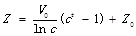for (1) and (3) and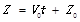for (2); Z0, V0 are initial values of Z and its rate respectively, when t = 0; c determines the change of V0 with age. Both these equations can be written in the joint differential form: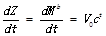(5)

Evaluation of the coefficients of (5) can be carried out using the recurrence formula similar to the Ford-Walford equation (4), which is usually used for estimation of Bertalanffy equation coefficients: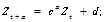(6)

V0 from (5) is calculated as V0 = (Z0 - d) ln(c), if c > 0 and c ≠ 1; V0 = d/m, if c = 1.

The plot of (6) in the coordinates Zt+m(Zt) is a vector whose start point has coordinates (Z1; Zm+1) and end point has coordinates (Ztm; Zt), where Z1, Zm+1 are the first and the second measurements; Zt-m, Zt are the penultimate and the last measurements respectively. Type of growth curve is determined by the position on the plot and the degree of slope of the vector (Fig. 1).

The values of the coefficients of (6) for infinite growth of the species of different taxa at different developmental stages are given in Table 1. Since the value of coefficient c depends on the units of age, and the value of coefficient d depends on the units of growth parameters, Tables 1 and 2 contain corresponding units used by authors of data. Fig. 2a-e shows the examples of approximation of infinite growth by (5).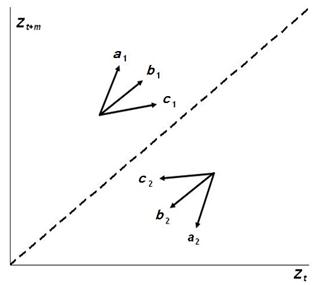Figure 1. Plots for (2). Dashed line is the bisector of the coordinate axes. Indexes: 1 - the increase of parameter Z; initial coordinates of the vectors lie above the bisector; 2 - the decrease of parameter Z; initial coordinates of the vectors lie below the bisector. Types of growth: a – an exponential (c > 1); b – a linear (c = 1); c – an asymptotic decelerating (c < 1).

Situation is more complicated in the case of finite growth. I believe that weight reduction occurring in this case after reaching a maximum determined by the spontaneous decay of the body, the rate of which is proportional to the body weight . Therefore, I introduced corresponding amendment in the calculation of the "pure" growth parameter: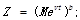(7)

v is the rate of spontaneous decay of the body. If v = 0 then we return to previous version of (5).

Table 1. Coefficients of (6) for infinite growth.

 Species Stage c d Data source Exponential growth type (c > 1) Bacillus megatherium Cell cycle 1.011±0.001 (min) 15±42 (nm)  Planorbarius corneus Larvae 1.037±0.006 (h) ‑12.1±6.5 (µm)  Leptestheria dahalacensis Larvae 1.050±0.015 (d) 0.72±0.23 (µg)  Pleurodeles waltl Larvae 1.028±0.001 (d) -0.38±0.41 (mm)  Parabolic (linear) growth type (с » 1) Planorbarius corneus Embryos 0.996±0.003 (h) 55±12 (µm)  Octopus bimaculoides Adults 1.001±0.001 (d) 0.30±0.06 (g)  Chirocephalus iaphanus Adults 0.999±0.036 (d) 0.99±0.15 (mm)  Heterozius rotundifrons Embryos 1.005±0.054 (d) 0.41±0.28 (mg)  Mamestra brassicae Caterpillar 0.997±0.023 (d) 0.28±0.13 (mg)  Ambystoma mexicanum Embryous 0.998±0.002 (h) 1.41±0.25 (mm)  Lacerta agilis Adults 1.00±0.02 (months) 0.39±0.03 (g)  Gallus gallus Embryos 1.01±0.02 (d) 0.15±0.02 (g)  Asymptotic decelerating (S-shaped) growth type (c < 1) Micrococcus sp. Cell cycle 0.975±0.099 (min) 265±5 (nm)  Lymnaea stagnalis Adults 0.884±0.005 (weeks) 4.41±0.16 (mg)  Planorbarius corneus Adults 0.937±0.014 (weeks) 0.60±0.12 (mg)  Margaritifera laevis Adults 0.922±0.013 (years) 0.83±0.09 (g)  M. margaritifera Adults 0.927±0.002 (years) 0.35±0.01 (mm)  Mytilus edulis Adults 0.942±0.017 (years) 7.61±0.67 (g)  Daphnia magna Adults 0.891±0.011 (d) 324±44 (µm)  Inahus dorsettensis Larvae 0.959±0.063 (d) 11.02±5.38 (mg)  Leptinotarsa decemlineata Larvae 0.970±0.021 (d) 0.33±0.09 (mg)  L. decemlineata Imago 0.753±0.040 (d) 3.46±0.24 (mg)  Blatella germanica Imago 0.895±0.003 (d) 0.55±0.07 (mg)  Apostichopus japonicus Adults 0.727±0.002 (years) 2.00±0.01 (g)  Clupea harengus Adults 0.748±0.003 (weeks) 1.94±0.03 (g)  Pleurodeles waltl Larvae 0.992±0.002 (d) 0.14±0.02 (g)  P. waltl Adults 0.960±0.001 (weeks) 0.10±0.01 (g)  Lacerta agilis Embryos 0.986±0.069 (d) 1.07±0.41 (mg)  Homo sapiens Embryos 0.988±0.004 (weeks) 0.52±0.04 (g) 

Note: The average values of the coefficients and standard error of the mean are shown; dimensions of the age and growth parameters are shown in parentheses; n is number of measurements.

Table 2. Coefficients of (6) for finite growth.

 Species c d v Data Source Exponential growth type (c > 1) Ambystoma mexicanum 1.051±0.005 (months) 0.18±0.06 (g) 0.105±0.002  Parabolic (linear) growth type (с » 1) Deroceras reticulatum 0.996±0.019 (weeks) 0.95±0.35 (mg) 0.040±0.001  Acheta domesticus 1.002±0.001 (d) 0.37±0.06 (mg) 0.0079±0.0001  Homo sapiens 0.995±0.001 (months) 0.59±0.03 (kg) 0.0003±0.0001  Asymptotic decelerating (S-shaped) growth type (c < 1) Enchytraeus albidus 0.925±0.008 (d) 0.36±0.06 (g) 0.0003±0.0001  Deroceras agreste 0.883±0.037 (weeks) 1.17±0.30 (mg) 0.017±0.008  Rattus norvegicus 0.687±0.015 (months) 4.68±0.09 (g) 0.0020±0.0002  Coturnix coturnix 0.956±0.002 (d) 1.48±0.03 (g) 0.0011±0.0002  Tyto alba 0.945±0.006 (d) 1.15±0.10 (g) 0.0013±0.0004 

Notes: The average values of the coefficients and standard error of the mean are shown; dimensions of the age and growth parameters are shown in parentheses; v is correction factor from (7). The data obtained for adults in all cases, besides A. mexicanum for which larvae (axolotl) was measured.

Values of the coefficients of (5) for finite growth of the species from different taxa at different developmental stages are shown in Table 2.

Fig. 2f shows an example of finite growth description by (5).

Some special cases have separate interest. Thus, if V0 = 0 or c = 0, the parameter Z is kept constant, i.e. the size of the body does not change. The plot of the function Zt+m(Zt) is represented by a point with coordinates (Z0;Z0).

If V0 < 0, the parameter of growth decreases. The plot of the function Zt+m(Zt) is the vector lying below the bisector (Fig. 1).

Thus, (5) and (6) can describe age related degradation of the weight. For example, weight loss during pupal stage of beetles Leptinotarsa decemlineata  is described by these equations with coefficients c = 0.981 ± 0.046; d = 0.07 ± 0.224; V0 = ‑4.16 ± 5.24 (number of measurements n = 18) (Fig. 3). For pupae of butterflies Bombix mori  c = 0.934 ± 0.023; d = 1.30 ± 0.34; V0 = ‑0.71 ± 0.46 (n = 20); for adults B. mori  c = 0.949 ± 0.030; d = 0.55 ± 0.19; V0 = ‑4.16 ± 5.24 (n = 11); for adults Mamestra brassicae  c = 0.936 ± 0.052; d = 0.85 ± 0.76; V0 = ‑0.048 ± 0.007 (n = 9); during cleavage stage of snails Planorbarius corneus (own data) c = 1; d = 5.3 ± 2.6; V0 = ‑2.80 ± 0.13 (n = 215). In all examples the age is measured in days from the larvae hatching, body weight is measured in mg, except the embryos of P. corneus for which age is measured in hours from first division, length is measured in µm.

The case with a negative coefficient c has separate interest. Such differential equations include wave components [49-51] associated with the appearance of an imaginary part in the value of the power factor kg = ln (c) from (1)-(3).

Endogenous biorhythms of specific growth rate have been identified and described for some species of gastropods and bivalves [12,13]. Such biorhythms are believed to be characteristic for the growth of a variety of animal taxa . Accounting of biorhythms allows more fully describe the process of growth. An example of such a description is shown in Fig. 3.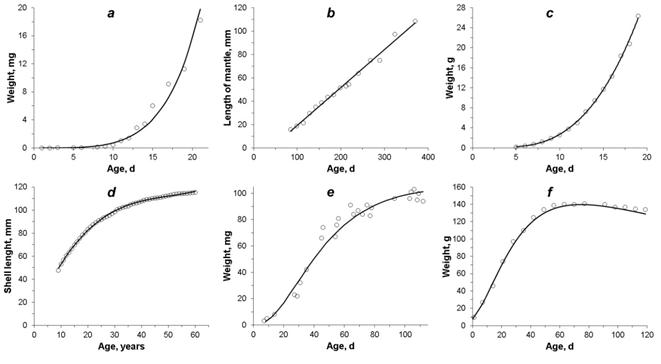Figure 2. Examples of growth curves and their approximation with united equation (5). Circles are experimental data. Curves are plots of the function (5). a - an exponential growth type; Leptestheria dahalacensis (Branchiopoda), larvae and juvenils ; b - a linear growth type; Octopus bimaculoides (Cephalopoda), adults ; c - parabolic growth type; Gallus gallus (Aves), embryos ; d - asymptotic decelerating growth type; Margaritifera margaritifera (Bivalvia), adults ; e - asymptotic S-shaped growth type; Blatella germanica (Insecta), adults ; f - finite S-shaped growth type; Coturnix coturnix (Aves); adults .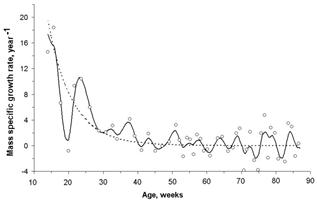Figure 3. The plot of the specific growth rate versus the age for Lymnaea stagnalis (Gastropoda) . Circles are experimental data. The dashed curve is the main trend describing by (5) with a positive coefficient c. The solid curve is the sum of the main trend and wave components, determined by the case of negative coefficient c.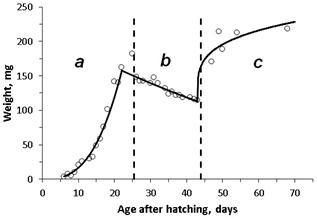Figure 4. Weight change during different developmental stages of the beetles Leptinotarsa decemlineata. Circles are experimental data . Vertical dashed lines are the borders of developmental stages: a - the larvae; b - the pupa; c - the imagoes. Solid line shows different growth curves in different developmental stages, approximated by (5) with different coefficients.

The equation (5) can be called the united growth equation, since it describes any type of animal growth observed in the nature. Type of growth is determined mainly by the value of the coefficient c: if c > 1, the dependence of Z(t) is exponential; if c = 1, the dependence is linear; if 0 < c <1 the dependence is asymptotic.

The existence of an equation capable to describe all known growth types indicates that for prolonged developmental stages the growth process seems to be in accordance with the physical and the chemical laws. Final result depends on the balance of the rates of assimilation, dissimilation and spontaneous disintegration of the biological system [1,3,4]. This does not mean that equation coefficients remain unchanged throughout the lifespan. Growth is too important process to leave it without control and regulation [8,9,52]. It is most likely that the regulation occurs over relatively small period of time by changing the rates of biosynthesis and reactions leading to disintegration of biomolecules. Hereafter, the process goes spontaneously until the moment when necessity of further regulation occurs. Such necessity may be connected with the achievement of a certain stage of development, the influence of external factors and/or the changes in physiological state.

Changing of growth type from stage to stage occurs in the majority of species. Thus, the birds’ embryos grow by parabolic type (Fig. 2c), whereas after hatching S-shaped finite growth type occurs (Fig. 2f).

Our data show that during embryogenesis of snails Planorbarius corneus stages with different types of linear growth sequentially replaced: decreasing of sizes (cleavage), constant sizes (blastula and gastrula), exponential growth (larvae stages), asymptotic or linear types of growth (postlarval stages) .

The sharpest change in the type of growth is observed during development of insects with complete metamorphosis (Fig. 4). Increase of larvae weight is replaced by weight degradation during the pupae stage. Weight of adults can increase  or decrease [24,47] due to species.

Nevertheless, at each developmental stage (5) is quite suitable to describe animal growth. Only the values of the coefficients are changed.

Power (allometric) dependence is often occurred between growth and a number of other biological parameters [4,53]:

Y= aXb;                    (8)

Y, X are measured parameters; a, b are coefficients.

Therefore, the equation (5) is suitable to describe any type of animal growth, as well as change of any biological parameter which is associated with body weight by allometric (power) dependence (8). For example, linear or weight sizes of organs, rate of metabolism etc. [53,54].

4. Conclusion

Proposed equation is very simple. It contains only two main coefficients and two additional coefficients for special cases of growth. Coefficients of (5) have quite clear biological interpretation. V0 determines the initial rate of the growth; c determines the character of growth rate change; b defines the impact of morphogenetic processes into growth process; v determines the rate of spontaneous decay of the body.

A small number of coefficients allow easily approximate the experimental data by (5). Presence of a linear form (6) allows comparative intrapopulational, interpopulation and interspecies analysis of the growth.

Acknowledgements

This work was supported by the Presidium of the Russian Academy of Sciences under the programs "Wildlife: Current Status and Problems of Development".

References

1. I. I. Shmalgauzen, "Growth and differentiation," in Rost zhivotnykh, S. Ya. Kaplansky, M. S. Mitzkevich, B. T. Tokin and I. I. Shmalgauzen, Eds. Moscow and Leningrad: Gosudarstvennoe izdatelstvo biologicheskoj i meditsinskoj literatury, 1935, pp. 74–84 (in Russian).
2. G. G. Vinberg, "Interrelation of growth and energy metabolism in poikilothermal animals," in Kolichestvennye aspekty rosta organizmov, A. I. Zotin, Ed., Moscow: Nauka, 1975, pp. 7–25 (in Russian).
3. L. von Bertalanffy, 1965. "On the von Bertalanffy growth curve," Growth, vol. 30, pp. 123–124.
4. M. V. Mina and G. A. Klevezal, "Animal Growth," Moscow: Nauka, 1976, 291 p. (in Russian).
5. A. I. Zotin, "Thermodynamic aspects of developmental biology," Monographs in Developmental Biology, vol. 5. Basel et al.: S.Karger, 1972, 159 p.
6. A. I. Zotin, "Quantitative growth theories (history, current state and prospects)," in Kolichestvennye aspekty rosta organizmov, A. I. Zotin, Ed., Moscow: Nauka, 1975, pp. 230–233 (in Russian).
7. A. I. Zotin and R. S. Zotina, "The phenomenological theory of development, growth, and aging," Moscow: Nauka, 1993, 364 p. (in Russian).
8. D. E. Gerrard and A. L. Grant, "Principles of animal growth and development," Dubuque, IA: Kendall/Hunt Publ. Co, 2003, 284 p.
9. K. L. Hossner, "Hormonal regulation of farm animal growth," Wallingford, Oxfordshire, UK; Cambridge, MA: CABI Publ., 2005, 223 p.
10. R. S. Zotina and A. I. Zotin, "Towards a phenomenological theory of growth," J. Theor. Biol., vol. 35, 1972, pp. 213–225.
11. R. S. Zotina and A. I. Zotin, "Integrated equations of growth," Zh. Obshch. Biol., vol. 34, 1973, pp. 606–616 (in Russian).
12. A. A. Zotin, "Patterns of growth and energy metabolism in the ontogeny of mollusks," Extended Abstract of Doctor of Sciences Dissertation, Moscow: IBR RAN, 2009, 30 p. (in Russian).
13. A. A. Zotin and S. Yu. Kleimenov, "Endogenous biorhythms of the specific growth rate in individual development of Lymnaea stagnalis (Lymnaeidae, Gastropoda)," Biology Bull., vol. 40, 2013, pp. 1–10.
14. A. I. Zotin, "Thermodynamics and growth of organisms in ecosystems," Can. Bull. Fish. Aquat. Sci., vol. 213, 1985, pp. 27–37.
15. E. Ford, "An account of the herring investigations conducted at Plymouth during the years from 1924-1933," J. Marine Biol. Assoc. U.K., vol. 19, 1933, pp. 305–381.
16. L. A. Walford, "A new graphic method of description the growth of animals," Biol. Bull., vol. 90, 1946, pp. 141–147.
17. A. A. Zotin, "Statistical estimation of allometric coefficients," Biology Bull., vol. 27, 2000, pp. 431–437.
18. A. A. Zotin and E. F. Kirik, "Growth of Planorbarius corneus (Gastropoda, Planorbidae)," unpublished.
19. T. I. Dobrynina, R. Ya. Bratchik, F. V. Monakov and E. B. Paveljeva, "Pecularities of growth, metabolism, and feeding of the clam shrimp (Conchostraca)," in Osnovy izuchenija presnovodnych ecosysytem, G. G. Vinberg, Ed ., Leningrad: ZIN AN SSSR, 1981, pp. 138–144 (in Russian).
20. S. G. Vassetzky, "The Spanish newt Pleurodeles waltlii," in Animal species for developmental studies, D. A. Dettlaff and S. G. Vassetzky, Eds., New York and London: Plenum Press, 1991. pp. 167–201.
21. J. W. Forsythe and R. T. Hanlon, "Effect of temperature on laboratory growth, reproduction and life span of Octopus bimaculoides," Mar. Biol., vol. 98, 1988, pp. 369–379.
22. P. S. Lake, "The effect of temperature on growth, longevity and egg production in Chirocephalus diaphanus Prevost (Crustacea: Anostraca)," Hydrobiologia, vol. 33, 1969, pp. 342–351.
23. H. H. Taylor and N. Leelapiyanart, "Oxygen uptake by embryos and oligerous females of two intertidal crabs, Heterozius rotundifrons (Belliidae) and Cyclograpsus lavauxi (Grapsidae): scaling and the metabolic costs of reproduction," J. Exp. Biol., vol. 204, 2001, pp. 1083–1097.
24. T. A. Alekseeva and N. D. Ozernyuk, "Dynamics of energy metabolism in ontogenesis of striped shield bug (Graphosoma lineatum L.) and cabbage moth (Mamestra brassicae L.)," Russ. J. Dev. Biol., vol. 42, 2011, pp. 73–78.
25. N. P. Bordzilovskaya and T. A. Dettlaff, "The axolotl Ambystoma mexicanum," in Animal species for developmental studies, D. A. Dettlaff and S. G. Vassetzky, Eds., New York and London: Plenum Press, 1991, pp. 203–230.
26. S. Yu. Kleimenov, "The intensity of energy metabolism in the early ontogeny of animals according to direct and indirect calorimetry data," Extended Abstract of Candidate of Sciences Dissertation, Moscow: IBR RAN, 1996, 21 p. (in Russian).
27. H. A. Murray, "Physiological ontogeny. A. Chicken embryos. 3. Weight and growth rate as function of age," J. Gen. Physiol., vol. 9, 1925. pp. 39–48.
28. A. A. Zotin, "Individual growth of Lymnaea stagnalis (Lymnaeidae, Gastropoda): II. Late postlarval ontogeny," Biology Bul., vol. 36, 2009, pp. 591–597.
29. A. A. Zotin and I. G. Vladimirova, "Respiration rate and species-specific lifespan in freshwater bivalves of Margaritiferidae and Unionidae families," Biology Bul., vol. 28, 2001, pp. 273–279.
30. A. A. Zotin and N. D. Ozernyuk, "Growth characteristics of the common mussel Mytilus edulis from the White Sea," Biology Bul., vol. 31, 2004, pp. 377–381.
31. G. E. Insarov, "Alternation of growth and reproduction in ontogenesis," in Energeticheskie aspekty rosta i razmnozheniya vodnykh bespozvonochnykh, L. M. Suschenya, Ed. Minsk: AN BSSR, 1975, pp. 23–31 (in Russian).
32. K. Anger, "Growth and elemental composition (C, N, H) in Inachus dorsettensis (Decapoda: Majidae) larvae reared in the laboratory," Mar. Biol., vol. 99, 1988, pp. 255–260.
33. L. I. Radzinskaya, I. S. Nikolskaya, T. A. Alekseeva, I. G. Vladimirova, P. I. Kovalenko and N. D. Ozernyuk, "Changes in the intensity of respiration during ontogenesis of some invertebrates," Ontogenez, vol. 34, 2003, pp. 317–320 (in Russian).
34. R. Walter and I. Lamprecht, "Modern theories concerning the growth equations," in Thermodynamics of Biological Processes, I. Lamprecht and A. I. Zotin, Eds, Berlin and New York: Walter de Gruyter, 1978, pp. 143–162.
35. Yu. E. Bregman, "The growth of sea cucumber (Stichopus japonicus) in Peter the Great Bay," Zool. Zhurnal., vol. 50, 1971, pp. 839–845 (in Russian).
36. A. Pütter, "Studien über physiologische Ähnlichkeit. VI. Wachstumsähnlichkeiten," Pflüger's Arch. ges. Physiol., vol. 180, 1920, pp. 298–304.
37. I. G. Vladimirova, S. Yu. Kleimenov and T. A. Alekseeva, "Dynamics of body mass and oxygen consumption in the ontogeny of the Spanish ribbed newt (Pleurodeles waltl): 2. Larval Stage," Biology Bul., vol. 39, 2012, pp. 10–14.
38. R. K. Danilov and T. G. Borovaya, "General and medical embryology," Sankt-Peterburg: SpetzLit, 2003, 231 p. (in Russian).
39. A. A. Zotin, "Equations describing changes in weight and mass-specific rate of oxygen consumption in animals during postembryonic development," Biology Bul., vol. 33, 2006, pp. 323–331.
40. I. G. Vladimirova, S. Yu. Kleimenov, T. A. Alekseeva and L. I. Radzinskaya, "Specific growth rate and the level of energy metabolism in the ontogeny of axolotl, Ambystoma mexicanum (Amphibia: Ambystomatidae)," Biology Bul., vol. 30, 2003, pp. 591–596.
41. A. A. Zotin, "Patterns of individual growth of gray garden slug Deroceras reticulatum," Biology Bul., vol. 34, 1978, pp. 457–462.
42. I. S. Nikolskaya, L. I. Radzinskaya and E. A. Prokofjev, "Change of respiration and wtight of cricket Acheta domesticus L. during growth and aging," Izvestiya Akademii Nauk SSSR, Seriya Biologicheskaya, No 4, 1986, pp. 628–633 (in Russian).
43. W. Thompson D'Arcy, "On growth and form," Cambridge: Univ. Press, 1942, 1116 p.
44. I. V. Ivleva, "Growth and reproduction of potworm (Enchytraeus albidus Heule)," Zool. Zhurnal, vol. 32, 1953, pp. 394–404 (in Russian).
45. A. A. Zotin, "Patterns of individual growth in the field slug Deroceras agreste (Gastropoda, Agriolimacidae)," Biology Bul., vol. 36, 2009, pp. 28–36.
46. L. R. Arrington, T. C. Beaty and K. C. Kelley, "Growth, longevity, and reproductive life of the Mongolian gerbil," Lab. Anim. Sci. vol. 23, 1973, pp. 262–265.
47. E. A. Prokofjev, "Quantitative analysis of growth and life span prediction," Extended Abstract of Candidate of Sciences Dissertation, Moscow: IBR RAN, 1983, 21 p. (in Russian).
48. C. C. White and L. I. Brisbin, "Estimation and comparison of parameters in stochastic growth models for barn owls," Growth, vol. 44, 1980, pp. 77–111.
49. A. I. Zotin and T. N. Vorobjeva, "Oscillatory processes in a steady state of nonlinear thermodynamic systems," in Kolebatelnye protsessy v biologicheskikh i khimicheskikh sistemakh. Vol. 2, E. E. Selkov, Ed., Pushchino-on-Oka: Institute of Biophysics, USSR Acad. Sci., 1971, pp. 120–124 (in Russian).
50. G. Nicolis and I. Prigogine, "Self-organization in nonequilibrium systems: from dissipative structures to order through fluctuations," New York: Wiley Interscience, 1977, 512 p.
51. A. B. Rubin, "Thermodynamics of biological processes," Moscow: Izdatelstvo MGU, 1984, 240 p. (in Russian).
52. A. I. Zotin, "Thermodynamic bases of biological processes: physiological reactions and adaptations," Berlin and New York: Walter de Gruyter, 1990, 293 p.
53. K. Schmidt-Nielson, "Scaling: why is animal size so important?" Cambridge: Univ. Press, 1984, 241 p.
54. A. I. Zotin and A. A. Zotin, "Direction, rates, and mechanisms of progressive evolution: thermodynamic and experimental bases)," Moscow: Nauka, 1999, 319 p. (in Russian).

 Contents 1. 2. 3. 4.
Article ToolsAbstractPDF(1443K)
Follow on us
PUBLICATION SERVICE
JOIN US
RESOURCES
SPECIAL SERVICES
ADDRESS
Science Publishing Group
548 FASHION AVENUE
NEW YORK, NY 10018
U.S.A.
Tel: (001)347-688-8931